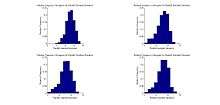# Plug-in principle

In statistics, the plug-in principle  is the method of estimation of functionals of a population distribution by evaluating the same functionals at the empirical distribution based on a sample.The best example of the plug-in principle, the bootstrapping method.

For example, when estimating the population mean, this method uses the sample mean; to estimate the population median, it uses the sample median; to estimate the population regression line, it uses the sample regression line.

It is called a principle because it is too simple to be otherwise, it is just a guideline, not a theorem.

1. Logan, J. David and Wolesensky, Willian R. Mathematical methods in biology. Pure and Applied Mathematics: a Wiley-interscience Series of Texts, Monographs, and Tracts. John Wiley& Sons, Inc. 2009. Chapter 6: Statistical inference. Section 6.6: Bootstrap methods